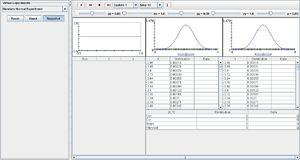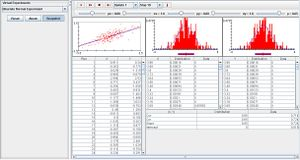# SOCR EduMaterials Activities BivariateNormalExperiment

## Description

The Bivariate Normal Experiment demonstrates the central tendency, variability, and spread of two quantitative variables in an event. A randomly generated point (X,Y) from a Bivariate normal distribution with mean (0,0) is graphically shown as a red dot in the scatter plot. The distribution regression line is also shown in the scatter plot as blue and the same regression line is shown as red. The middle graph contains the distribution graphs that display the density function and moments of X and the last graph displays the same information for variable Y. For each update, the coordinates are recorded in the data table to the left and the last two tables give the numerical moment values of X and Y, as well as the distribution standard deviation and mean. The last table gives the distribution and sample correlation.

## Goal

To provide a simple applet that provides information for bivariate data that consists of two quantitative variables (e.g. age, height, blood pressure, etc.) and build a common ground about these events while enforcing the knowledge of bivariate procedures.

## Experiment

Go to the SOCR Experiments [] and select Bivariate Normal Experiment from the drop-down list of experiments located at the top left. The image below shows the initial view of this experiment:When pressing the play button, one trial will be executed and recorded in the distribution table below. The fast forward button symbolizes the nth number of trials to be executed each time. The stop button ceases any activity and is helpful when the experimenter chooses “continuous,” indicating an infinite number of events. The fourth button will reset the entire experiment, deleting all previous information and data collected. The “update” scroll indicates nth number of trials (1, 10, 100, or 1000) performed when selecting the fast forward button and the “stop” scroll indicates the maximum number of trials in the experiment.

The distribution standard deviations and correlations can be varied with the scroll bars above the graphs. Note that modifying the mean and standard deviation of X and Y only changes the spread of the distribution graphs for X and Y. The distribution regression line (blue) in the scatter plot will remain horizontal and the density function graphs of X and Y will continue to be normal. When varying p, only the slope of the distribution regression line in the scatter plot changes; when p>0.50, the slope is positive and when p<0.50, the slope becomes negative. The image below displays information when p>0.50 after many trials.## Applications

The Bivariate Experiment is a special case for values that X and Y that are finite. If either of the values is infinite, then the bivariate probability reverts to the univariate probability. This SOCR Java applet could be used in many different kinds of studies. For instance, a professor may use the SOCR Bivariate Experiment to determine the grades for his students since the bivariate normal probability offers a “bell-shaped” curve. Another example may be to study the population in a big city’s subway throughout a weekday. The distribution graph may be centered at the time of peak hours in the subway. Using the SOCR Bivariate Normal Experiment will display the exact mean and standard deviation of the population in the subway on a daily basis.